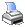﻿ MCL Variables in MCL Functions > Introduction to MCL Variables
 Navigation:  MCL Variables in MCL Functions > Introduction to MCL VariablesYou can embed variables in MCL functions. They will be replaced with either the default values or the values that you supply before the functions are evaluated.

How to Define an MCL Variable and Its Default Value

An MCL variable is a number enclosed by a pair of quotes { and }. The number can be 1 to 9. For example {1} means variable number 1. You can put an optional default value by separating the variable number and the default value with a ":". For example {3:MyValue} defines a MCL variable {3} with a default value MyValue.

MCL Variables of a View is Independent of MCL Variables of other Views

MCL variables of a view is independent of MCL variables of other views. You change the value of {2} in view X will not affect the value of {2} of view Y.

Rules for Default Values of MCL Variable

It is a good practice to always set a default value for an MCL variable. The default value should be set in the first occurrence of the MCL variable.

MCL Variables in Starter Views and History/Statistics Views

You can use MCL variables in both starter views and history/statistics views. However they are 2 independent sets of variable and have no relations with each other. Even if you have defined a value for {1} in a starter view, the value of it will not automatically passed to the history/statistics view. However you can pass its value to a history/statistics view via the rules discussed on the topic Using MCL Variables As View Arguments.

Examples

HrActivity({3})

In the above function, MCL variable {3} is defined. Since there is no default value, you must later supply it with a value.

HrActivity({2:28})

In the above function, MCL variable {2} is defined with a default value 28. If no value is supplied to the variable, the function is equivalent to HrActivity(28).

JrFp(,"For HrHorse()='{1}'")

If you later set {1}=Brilliant Chapter, the above function will be equivalent to JrFp(,"For HrHorse()='Brilliant Chapter'")